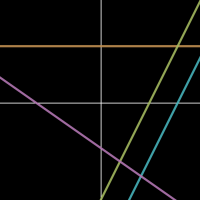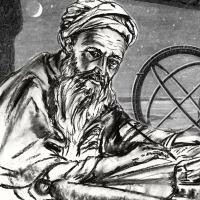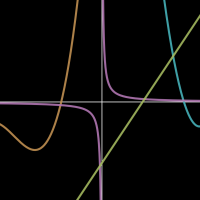# Mathematics

Sort By:

MathematicsIn this course, Professor Keith Ball (University of Warwick) gives an introduction to graphs, covering topics A8-A10 in the Pearson Edexcel GCSE (9-1) Mathematics (1MA1) Specification for Foundation Tier. In the first mini-lecture, we provide...

7 lectures

0:50:00

Prof. Keith Ball

Warwick University

MathematicsIn this course, Professor Kevin Buzzard (Imperial College London) explores numbers, in particular the counting numbers and their abstract nature. In the first mini-lecture, we consider what ‘2 + 2 = 4’ really means and introduce the idea of an...

8 lectures

0:55:30

Prof. Kevin Buzzard

Imperial College London

MathematicsIn this course, Professor Keith Ball (University of Warwick) explores solving equations, covering Topics A17-A18 in the Pearson Edexcel GCSE (9-1) Mathematics (1MA1) Specification for Higher Tier. In the first mini-lecture, we give an introduction...

7 lectures

1:16:35

Prof. Keith Ball

Warwick University

MathematicsIn this course, Professor Keith Ball (University of Warwick) gives an introduction to graphs, covering topics A8-A10 in the Pearson Edexcel GCSE (9-1) Mathematics (1MA1) Specification for Higher Tier. In the first mini-lecture, we provide...

7 lectures

0:58:28

Prof. Keith Ball

Warwick University

MathematicsIn this course, Dr Piers Bursill-Hall (University of Cambridge) explores the origins of algebra: Hindu-Arabic arithmetic. In the first mini-lecture, we consider types of arithmetic used by ancient peoples, including Egyptians, Mesopotamians, and...

7 lectures

1:15:52

Dr Piers Bursill-Hall

Cambridge University

MathematicsIn this course, Professor Keith Ball (University of Warwick) explores solving equations, covering Topics A17-A18 in the Pearson Edexcel GCSE (9-1) Mathematics (1MA1) Specification for Foundation Tier. In the first mini-lecture, we give an...

4 lectures

0:41:54

Prof. Keith Ball

Warwick University

MathematicsIn this course, Professor Keith Ball (University of Warwick) continues exploring graphs, covering Topics A11-A13 in the Pearson Edexcel GCSE (9-1) Mathematics (1MA1) Specification for Higher Tier. In the first mini-lecture, we introduce quadratic...

8 lectures

1:24:05

Prof. Keith Ball

Warwick University

MathematicsIn this course, Professor Keith Ball (University of Warwick) continues exploring graphs, covering Topics A11-A12 in the Pearson Edexcel GCSE (9-1) Mathematics (1MA1) Specification for Foundation Tier. In the first mini-lecture, we introduce...

4 lectures

0:33:55

Prof. Keith Ball

Warwick University

MathematicsIn this course, Professor Keith Ball (University of Warwick) continues his exploration of solving equations, covering Topics A19 and A21 in the Pearson Edexcel GCSE (9-1) Mathematics (1MA1) Specification for Foundation Tier. In the first...

5 lectures

0:49:15

Prof. Keith Ball

Warwick University

MathematicsIn this course, Professor Keith Ball (University of Warwick) continues his exploration of solving equations, covering Topics A19-A21 in the Pearson Edexcel GCSE (9-1) Mathematics (1MA1) Specification for Higher Tier. In the first mini-lecture, we...

7 lectures

1:12:19

Prof. Keith Ball

Warwick University